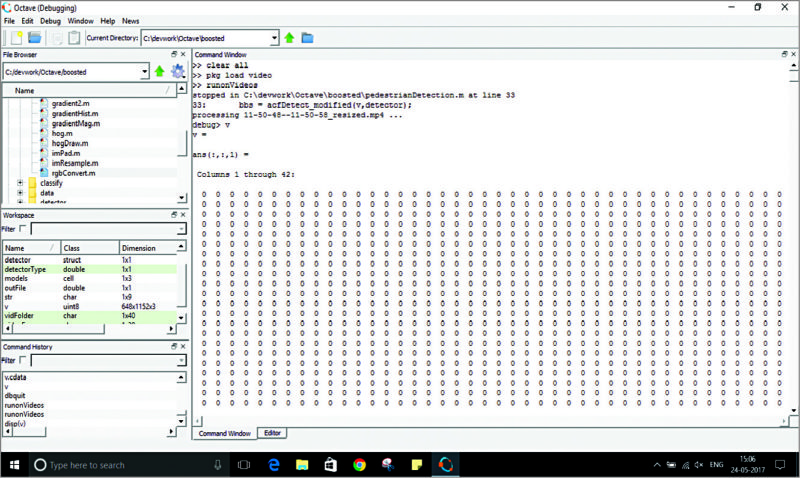# Ease Your Numerical Analysis with Octave 4.2.1

5675

To calculate the area of a circle, you can follow the simple formula of πr2 (where ‘r’ is the radius of the circle). But, calculating the mathematical value of ‘π’ beyond nine digits involves a technique called numerical analysis. When designing complex products such as aircrafts or rockets, Octave 4.2.1 can be a boon for designers.

Octave is a special high-level software used for numerical computation. It is basically a solution provider to linear and non-linear numerical problems.

## Numerical Analysis with Scientific Octave and how it works

Octave is a powerful scientific programming language that runs on GNU/Linux, MacOS, BSD and Windows. Users can solve all the linear/non-linear algebra and statistical analysis and other numerical experiments using Octave.

On most systems, Octave runs with the shell command ‘octave,’ where it displays a graphical user interface.

Octave can do elementary calculations, create a matrix, use integral differential equations, produce a graphical output, edit and document all the processes. Each of these calculations involves a set of programs. For example, to verify Euler’s identity, you need to follow the simple steps given below:
ei×pi=–1
octave:1> exp (i×pi)

Besides, Octave has a help facility where documents in printed form are also available in Octave prompt as creation files for both are the same. With Octave, users can recall, edit and re-issue previous commands. They can specify the program to run, which Octave calls from its set of programs. And while Octave is running, until the terminal command is used, users can’t exit the program.

## What is new in version 4.2.1

Basically, this version has been released for bug fixing. But apart from all the similarities that it shares with MATLAB, version 4.2.1 is different in that it does not print outputs to the console until all the commands in queue are terminated. This function is controlled by a Boolean variable.

Besides, there were several bugs in Octave’s previous version, which have been fixed in 4.2.1.

Plotting in Octave is done through OpenGL function, which is displayed as an invalid function while zooming in this software. This bug has been fixed in the recent version.

Earlier, plot code and source code were mismatched, so proper documentation was difficult. Suppose if there was a manual to be compiled, the histogram for this purpose used to be different each time. This affected the documentation. The 4.2.1 version resolves this issue.

There are some other small but time-saving changes that fix the incorrect handling or mismanaged binary data fed in complex constants. Also, as soon as the user exits Octave, the editor saves the widget settings again.

Users experienced desktop crash or instant logout when using Octave in Xubuntu 16.04. Version 4.2.1 resolves this issue, too.

Besides, there are many other bugs related to commands, which have been fixed.

## Applications

Apart from use on desktops for personal scientific computing, Octave is also used by researchers and industries. In addition to numerical analysis, it is majorly used for signal processing using FFT techniques. Signal processing is a major application area in digital electronics, where it is an integral function of test and measurement instruments like spectrum analysers, oscilloscopes and frequency meters.

Above all, digital signal processing and audio signal processing are also carried out using Octave software for proper acquisition of images and audio samples. To simply the process, Octave considers its audio data as vectors of samples.Fig. 4: Image processing in Octave (Image courtesy: https://stackoverflow.com)

## In nutshell

Although Octave is compatible with MATLAB in many aspects and shares many similarities, there are fields where MATLAB dominates Octave, and vice-versa.

But, above all, Octave is an open source software that finds many uses and is gaining popularity over a period of time with new released versions.